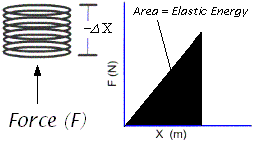### Elastic Energy of SpringsIn order to compress a spring an external force needs to be applied.

The force F will compress the spring by an amount (-x)

The amount of work done to compress the spring is now the Elastic Potential Energy stored in the spring.
If the spring is allowed to spring back to its original position it will release its stored energy as useful work.

This amount of work is best calculated by taking the area under the F-graph.

Area = 1/2 (base x height)  ... The area of a triangle
= 1/2 (F) (
x)

But F = kx .... from Hooke's Law

Therefore  A= 1/2(k)(x)2

This represents the stored energy in the spring or the potential elastic energy (Ee) of the spring.

Ee =1/2(k)(x)2